# What Is an Algorithm?

Description

Participants will understand what an algorithm is, why algorithms matter, and how algorithms are used in both everyday life and computer science.

Materials

• [One per participant, and one for the educator] Handout: What Is an Algorithm?

• [One per group of two participants] Handout: Recipe [educator version and participant version]

• [One per participant] Handout: Algorithms in Prime Time [educator version and participant version]

• Projector and projection screen

• Flip chart (or poster) [If a board is not available]

• Marker

• A set of eight large, white paper cups with the following numbers drawn in black marker on them (one number per cup): 5, 23, 28, 37, 64, 98, 110, and 125. [The numbers should be drawn a) on both sides of the cup, so that the educator can see one side, and the participants can see the other, and b) so that participants in the room will be able to see the numbers.]

• [One per participant] Paper

• [One per participant] Pens or pencils

• Printout of Muḥammad ibn Mūsā al-Khwārizmī statue image

• Printout of bubble sort images — Bubble sort #1, bubble sort #2, bubble sort #3, bubble sort #4, bubble sort #5, bubble sort #6, and bubble sort #7

• Printout of merge sort diagrams — Step One: Divide, and Step Two: Conquer

Resources

Activity #1: Algorithm Fundamentals

SAY:

• Have you ever wondered how your favorite restaurant makes your favorite dish perfectly every time? Or how Siri or Alexa can respond to a question you’re asking? How self-driving cars work? Believe it or not, all these have one thing at their core: algorithms.

• An algorithm is a clearly given set of step-by-step instructions to solve a problem or accomplish a task.

[Project this definition, the first bullet point on the What Is an Algorithm? Handout, on a projection screen. Pass out the What Is an Algorithm? Handout.]

SAY:

• Algorithms might be expressed in words, computer programs or code, or even as recipes. Some algorithms may be simple routines, directions, or processes you use in your daily life. For example, to wake yourself up in the morning, you might get out of bed, go to the bathroom and turn the water faucet to cold, let it run for 30 seconds, splash your face with water three times, and pat your face dry with a towel.

• Note here that these are steps to accomplish a task, and they are very clear and detailed. For instance, the amount of time the faucet is turned on and the number of times you splash your face with water are noted.

• Another example of an algorithm is a recipe for your favorite cookies where the instructions are clearly given, step-by-step.

• But not all routines / recipes are algorithms. We’ll get to this point soon!

• You can probably think of many types of routines you do everyday that accomplish different tasks. Can anyone share an example of steps to one of your routines?

[Examples could include, for instance, the directions needed in getting from their house to their friend’s house, the sequence of warm-up exercises they follow before taking a run, or the set of steps involved in washing their clothes. As participants share their routines, ask participants if they feel their steps are clear / precise. ]

SAY:

• You have actually worked with algorithms in every math class you’ve taken.

• In school, you learned algorithms for addition, subtraction, multiplication, and division.

• In geometry, you calculate areas of geometric shapes using algorithms.

• For example, when you calculate the area of a rectangle, you use a simple algorithm that multiplies the length of the rectangle by the width of the rectangle.

• Can you think of other instances from math where you used an algorithm to solve a mathematical problem?

[Possible examples include adding and multiplying fractions (e.g., 3 4 + 18 = 68 + 18 = 78) and / or solving equations (e.g., 3x + 2 = 5).]

SAY:

• The concept of an algorithm has been around for a very long time.

• In fact, the world’s first known mathematical algorithms were created by the Babylonians on clay tablets nearly 4,000 years ago.

• The word “algorithm” itself originated from the name of a 9th-century Persian mathematician, Muḥammad ibn Mūsā al-Khwārizmī (a pronunciation may be found during 0:18 - 0:20 of the following video), known for his work on explaining step-by-step procedures for solving mathematical problems.

[Feel free to project the image of a statue of Muḥammad ibn Mūsā al-Khwārizmī, (in Latin, “Algoritmi”), in his birthplace of Khiva, Uzbekistan, below, on a projection screen.]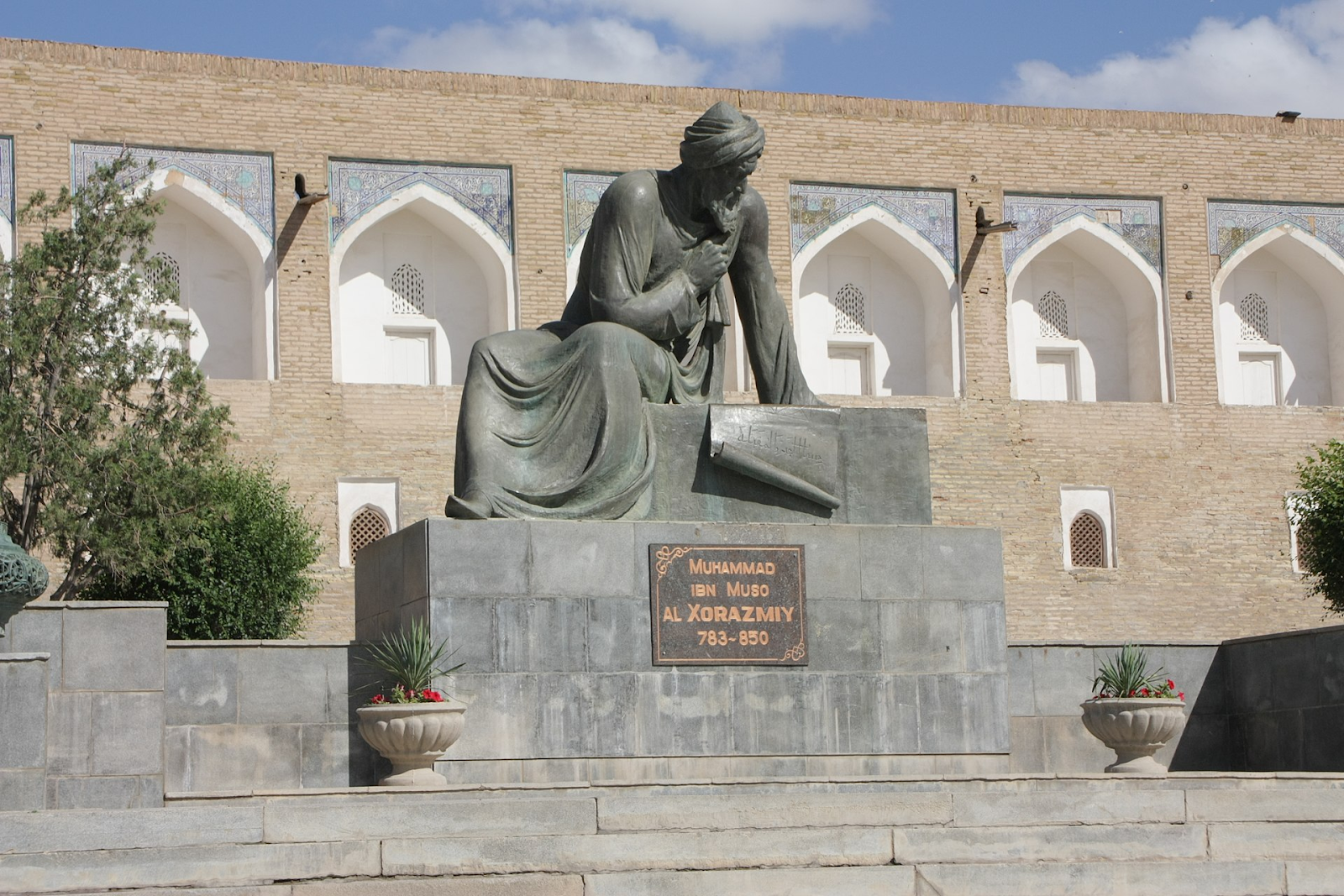Statue of Muḥammad ibn Mūsā al-Khwārizmī image

SAY:

• In today’s world, algorithms are everywhere. They are in our mobile devices, laptops, automobiles, appliances, and even toys!

• These algorithms are computer algorithms.

• In computer science, an algorithm is a sequence of precise instructions that tell a computer how to solve a problem or accomplish a task.

[Project this definition, the second bullet point on the What Is an Algorithm? Handout, on a projection screen.]

SAY:

• So, we can see that the definition of an algorithm and the definition of a computer algorithm are basically the same. The main difference is that computer algorithms run on computers.

• Let’s take a closer look at algorithms by exploring their characteristics and properties.

• We’ll do this by looking at an algorithm represented by a recipe, with the understanding that the characteristics and properties we will examine also apply to computer algorithms.

• This is a recipe that would be really fun to make for you and your friends!

[Pass out the Recipe: Participant Handout. Organize participants into pairs. In pairs, have participants discuss: Is this recipe an algorithm? Why or why not? Give them 10 minutes to do this. Remind participants that the steps need to be clearly stated so that anyone would be able to make this recipe.]

• Let’s come back together. What did you determine? Do you think the recipe is an algorithm? Why or why not?

[The recipe is not an algorithm for two key reasons. First, some steps are ambiguous (e.g., in the first step, the amount of time specified to cook the black bean mixture is not provided). Additionally, for some of the ingredients, the specific amounts needed are not given. Second, as we can see from the fifth step, the recipe results in six tacos, not the eight that the goal asks for. The process explained in the steps is also not as efficient as it could be — step two asks the reader to step away from the recipe and watch a TV show for a half-hour. As we will soon learn, while an algorithm’s efficiency isn’t an absolute requirement, it is ideal and, in some cases, can be critically important.]

SAY:

• Let’s take a look at what this recipe looks like as an algorithm!

[Project the recipe on the Recipe: Educator Handout on a projection screen.]

SAY:

• Now, it’s important to note that there are several key characteristics of an algorithm.

[Write down the following bolded characteristics on a flip chart / poster or board.]

SAY:

• First, the goal of the algorithm needs to be well-defined. For example, if the goal in our recipe example had been “Make a bunch of tacos,” we would not know how to accomplish this goal.

• Second, the step-by-step instructions need to be clearly given. If the recipe on your handout had been an algorithm, you would be able to give it to someone else, and they would know the exact amount of ingredients needed and the steps involved in correctly preparing the tacos, like how long to cook the black bean mixture.

• However, there is some flexibility in following a recipe. For instance, the time needed to cook the black bean mixture might vary, depending upon whether or not the person who is cooking it think it’s ready (e.g., based on the mixture’s consistency). So, the recipe might more appropriately give a range of time for cooking the bean mixture. Unlike us, however, a computer algorithm needs to follow a precise set of instructions to accomplish a task.

• And third, the algorithm should be correct. The recipe on your handout asks for 12 cans of beans. This is a misprint or error in the recipe because it would mean each taco would get over a can of black beans! Also, the recipe on your handout resulted in six tacos. This is incorrect since the goal at the top asks for eight tacos (I think the tacos were so delicious that the person who made them ate two before serving them!).

• We can think of the number of servings of tacos and the corresponding measured ingredients as the inputs in our recipe example. Following the steps of the recipe (as shown on the Recipe: Educator Handout) results in eight delicious tacos — our output. But, what if we want to make nine tacos? Do we have to write an entirely new algorithm?

[No, we can easily adjust the amount of ingredients  so that we can input any number of servings, from an individual serving to eight, nine, 20, or as many as needed!]

SAY:

• And, while the efficiency of an algorithm isn’t absolutely necessary, in certain situations, it can be critically important. Broadly, efficiency describes situations where resources such as materials, labor, or time are used well, without wasting them. Efficiency in the context of computer algorithms typically refers to the time taken to complete the algorithm or the space (i.e., memory) the algorithm takes up on a computer. But, in the taco recipe on your handout, we see an inefficient use of resources (i.e., money and storage) when we see that the ingredients call for 12 cans of black beans. Only two cans are needed to make the recipe. The extra ten are a waste of money and storage space (unless black beans are your favorite food!).

• For a recipe, efficiency would be particularly important for the main chef of a popular and busy restaurant! And in the recipe example on your handout, taking a break for 30 minutes to go watch your favorite TV show not only adds more time to the preparation, but also, in this case, might be disastrous, as the bean mixture is sitting on the stove cooking for a half hour.

• You’ll also notice at the top of the recipe I have an output, or a goal — that the recipe results in eight tacos.

SAY:

• In translating these characteristics to computer algorithms, let’s look again at how we describe algorithms that run on computers. [Read the second bullet point on the What Is an Algorithm? Handout.]

[Project this definition, the second bullet point on the What Is an Algorithm? Handout, on a projection screen.]

SAY:

• Another way to view computer algorithms is [Read the third bullet point on the What Is an Algorithm? Handout.]

[Project this definition, the third bullet point on the What Is an Algorithm? Handout, on a projection screen.]

SAY:

• As we just learned, a desirable feature of an algorithm is that it is efficient, which, among other things, includes the time it takes for the computer to run the algorithm.

• But, essential to computer algorithms is that the problem / task is well-defined, and  the step-by-step instructions are precisely given and are implementable by a computer.

• Computer algorithms can’t run on vague goals and instructions.

• The nature of computer algorithms (as seen in the third definition on your What Is an Algorithm? Handout) is that an input, or set of inputs, relevant to the goal of the algorithm, produces, by way of a sequence of implementable computer instructions, an output, or outputs, that attempt to solve the problem correctly.

• Here are some examples of computer algorithms that you might encounter.

• When you’re riding in a car, an array of computer algorithms are at work. For example, sensors detect input data such as miles traveled and gas consumed, and calculate gas mileage (i.e., the output).

• When you enter a destination on your GPS (the input), the GPS system produces an output of the shortest route.

• And when you ask Siri or Alexa a question (the input), these systems (hopefully!) produce a helpful response (the output).

• When you go online to search for something on a search engine, like Google, your input text produces an output of the most relevant websites.

• Today, computer algorithms are everywhere. They are in our mobile devices, laptops, cars, and everyday appliances.

• Algorithms — whether helping you make your favorite cookies or responding to your online searches — are all around us.

Activity #2: Out of Order – Algorithms to the Rescue!

[Assemble the white numbered cups in the following order on the table in front of you, from first to last: 64, 23, 5, 98, 125, 110, 28, and 37.]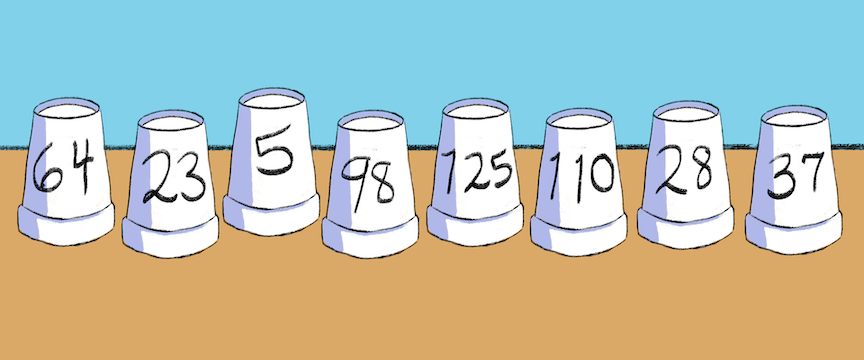Bubble sort image #1

SAY:

• Let’s explore a specific type of algorithm, called a sorting algorithm.

• “Sorting” typically refers to putting a list of items in numerical or alphabetical order.

• There are many examples of instances where having things in a specific order is really important, like in a mobile phone’s contact list.

• Can anyone think of other examples?

[Other examples might include the index of a book, dictionary entries, or books in a library.]

SAY:

• Sorting algorithms are a fundamental family of algorithms in computer science with a wide range of applications.

• When the World Health Organization, for example, collects incidences (i.e., rates) of a certain disease across hundreds of regions around the world, computer sorting algorithms systematically organize the data in increasing or decreasing order for tables, graphical representations, and data analysis.

• Let’s consider some more examples of sorting algorithms.

• Every time you search online using a search engine, such as Google, your search is processed by many sophisticated algorithms, with sorting algorithms built into the process.

• And when you go online to find the most popular pizza restaurant near you, computer algorithms, on websites like Trip Advisor and Yelp, sort these pizza restaurants by proximity and ratings provided by users of the website.

SAY:

• Let’s look at two computer sorting algorithms.

• One is known as the bubble sort.

• And the other is a merge sort — a sort based on a divide and conquer strategy.

SAY:

• [Project the following definition of a bubble sort on a projection screen.] A bubble sort is a simple sorting algorithm that repeatedly goes through a list of items, compares adjacent items, and swaps them if they are not in the correct order. This process is repeated until the items are sorted.

• I will now demonstrate the bubble sort with these eight numbered cups in front of me.

• My goal will be to sort these cups in increasing order.

SAY:

• Let’s compare the first two cups — 64 and 23.

• They are out of order — 23 is less than 64. So, these two cups should be swapped so that 23 comes before 64.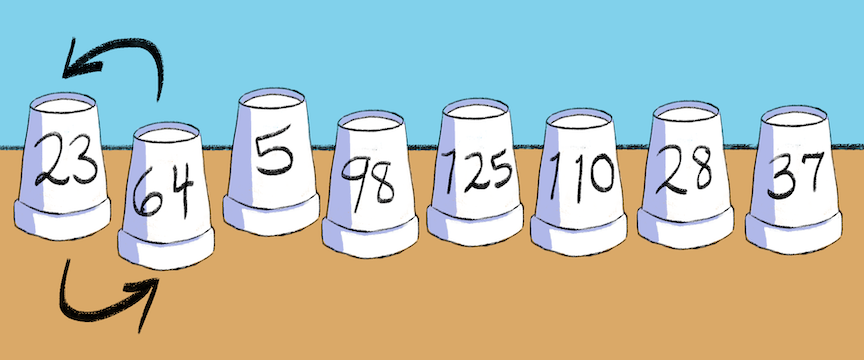Bubble sort image #2

SAY:

• Now, let’s look at the next adjacent pair of cups, numbered 64 and 5. Since 5 is less than 64, the cups are out of order, so I’ll swap them.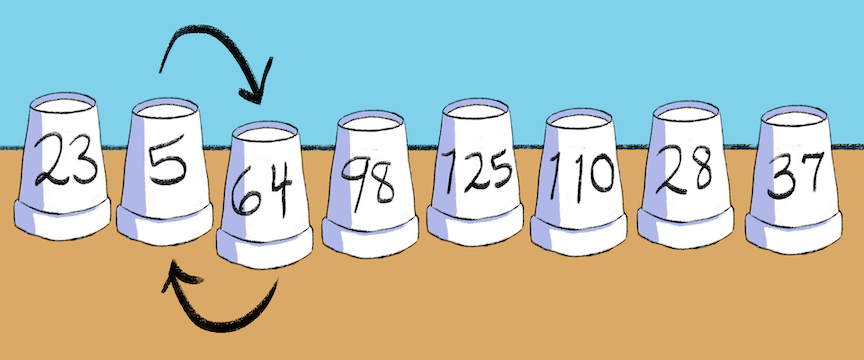Bubble sort image #3

SAY:

• Next, let’s compare the adjacent pair of 64 and 98. Since the pair is not out of order, I won’t swap these two.

• Then, I’ll compare the adjacent pair of 98 and 125. As before, since the pair is not out of order, I won’t swap these cups.

SAY:

• Continuing in this way with the next adjacent pair, cups numbered 125 and 110, I’ll swap them.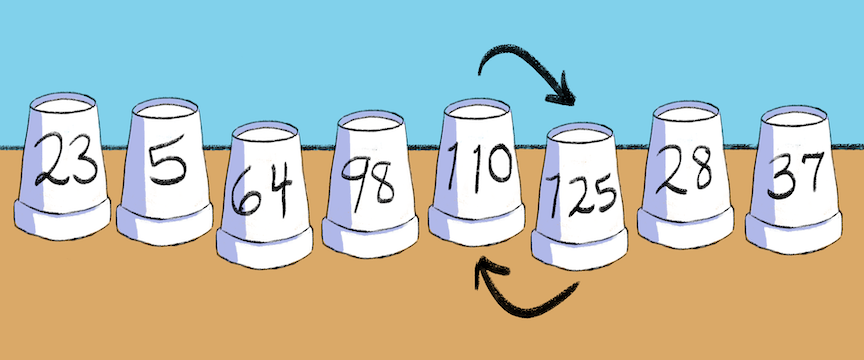Bubble sort image #4

SAY:

• Then, I’ll swap cups numbered 125 and 28.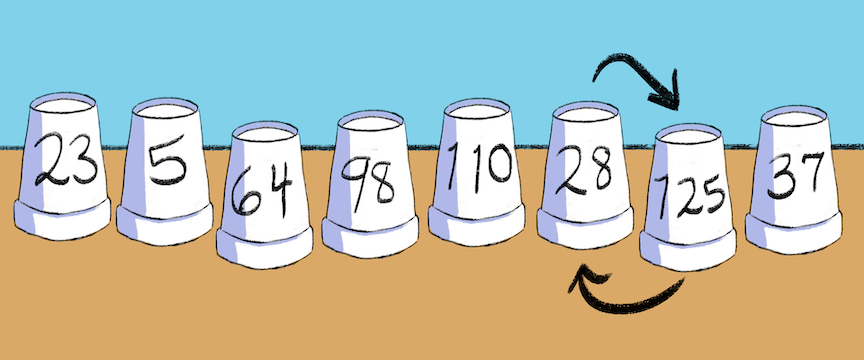Bubble sort image #5

SAY:

• And, finally, I’ll swap the pair of cups 125 and 37.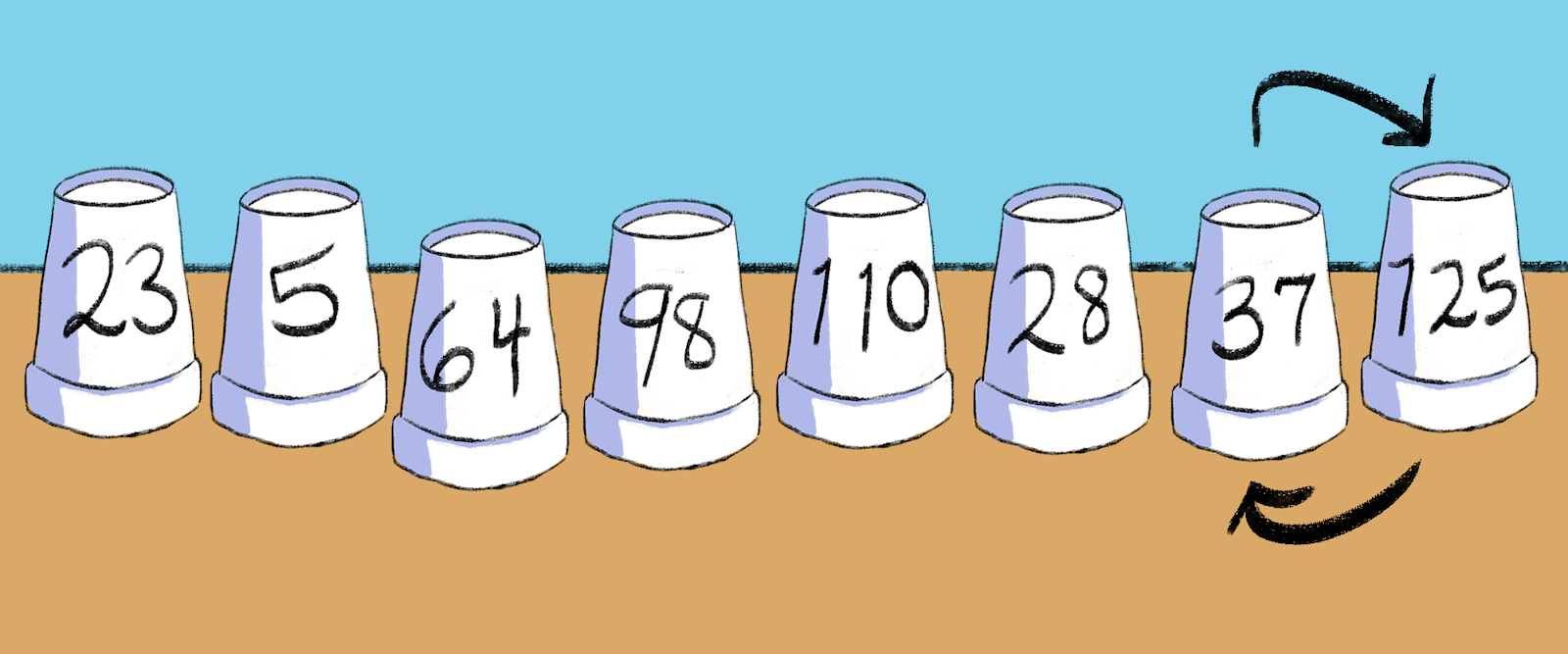Bubble sort image #6

SAY:

• We can see that the cups are still not in increasing order. But, let’s note a few things.

• First, I’ve made seven comparisons of pairs of numbers.

• Second, the largest numbered cup in this list, 125, has moved to the end of the row, where it should be. This number has bubbled up to the end of the list.

SAY:

• I’ll repeat this process by going back to the first two cups in the row, numbered 23 and 5.

• This time, let’s compare the cups together — deciding between swap or no swap.

[As you move along the row, ask participants if they think you should or should not swap the cups by asking, “Swap or no swap?” Going through a second time gives the following arrangement of cups: 5, 23, 64, 98, 28, 37, 110, and 125. Note to participants that, this time, we only had to make six comparisons, because we knew, from the first round of comparisons, that 125 was the largest number. So, we didn’t need to compare 110 and 125.]

• Do you see what we have achieved in this second round of comparisons?

[The cups are still out of order, but the second largest number (110) is now right before the largest number, 125, as it should be.]

SAY:

• Since the cups are still out of order, we need to go through the row again. Once again, we start at the beginning, with cups numbered 5 and 23.

[As you move along the row, ask participants if they think you should or should not swap the cups by asking, “Swap or no swap?” Going through a third time gives the following arrangement of cups: 5, 23, 64, 28, 37, 98, 110, and 125.]

• Do you see why we only needed to make five comparisons in the third round?

[Only five comparisons were made because, from the second round, we knew that the two largest numbers in the set (110 and 125) were correctly positioned at the end. So, we didn’t need to compare 98 and 110, or 110 and 125.]

SAY:

• We’re making progress, but the cups still aren’t in increasing order.

• I know this seems like it’s taking forever! But, computers are much faster than people, and we’ll see a faster way to order these numbers very soon!

• Now, let’s go through the row yet again using the same process. As before, we’ll return to the beginning of the row, with cups numbered 5 and 23.

[As you move along the row, ask participants if they think you should or should not swap the cups by asking, “Swap or no swap?” Going through a fourth time gives the following arrangement of cups: 5, 23, 28, 37, 64, 98, 110, and 125. Note to participants that, this time, we only made four comparisons. From the third round, we knew that the three largest numbers in the set (98, 110, and 125) were correctly positioned at the end. So, we didn’t need to compare the pairs 64 and 98, 98 and 110, and 110 and 125.]

SAY:

• Now the cups are in increasing order!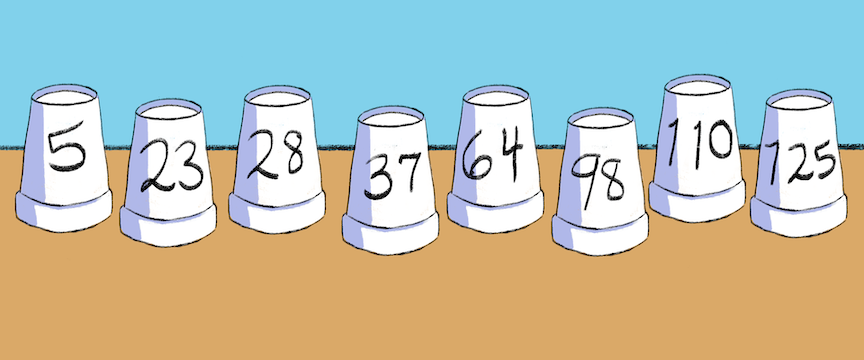Bubble sort image #7

• What do you think of the bubble sort in terms of the amount of work it involved?

[One possible answer is that while the bubble sort is simple, it can be very time-consuming with all the comparisons made.]

SAY:

• This sort involved 22 comparisons [calculated by totaling 7 comparisons (first round) + 6 comparisons (second round) + 5 comparisons (third round) + 4 comparisons (fourth round).]

• Let’s suppose each comparison takes one second. Then, the time it would take to complete this sort would be 22 seconds.

• But, now, let’s suppose someone handed us a list of 1,000 numbers to sort in increasing order.

• In this case, the bubble sort might require close to half a million comparisons. This would take us 5.8 days to complete — without taking any breaks!

• Running this algorithm on a computer, however, with an unsorted list of eight numbers (as in our example), would order the list almost instantaneously!

• But, with an unordered list of 1,000 numbers, the algorithm would take a few  minutes, which is actually a long time for a computer.

SAY:

• The bubble sort is simple but not efficient.

• Many other computer algorithms sort faster and more efficiently.

• One of these is the merge sort.

[Project the two bullet points below, under “SAY,” on a projection screen.]

SAY:

• The strategy behind the merge sort is divide and conquer — an approach used in many computer algorithms, but also used to solve problems in general.

• The idea behind divide and conquer is to make a problem easier to solve by dividing the problem into simpler versions of itself, solving these simpler versions, and then combining the versions to solve the problem you started with.

SAY:

• Let’s illustrate the merge sort with our eight numbered cups.

[Rearrange the cups again so that the numbered cups are presented in the same order at the start of this activity: 64, 23, 5, 98, 125, 110, 28, and 37.]Bubble sort image #1

SAY:

• The first part of the merge sort is “divide.”

[Project the Merge Sort — Step One: Divide diagram on a projection screen.]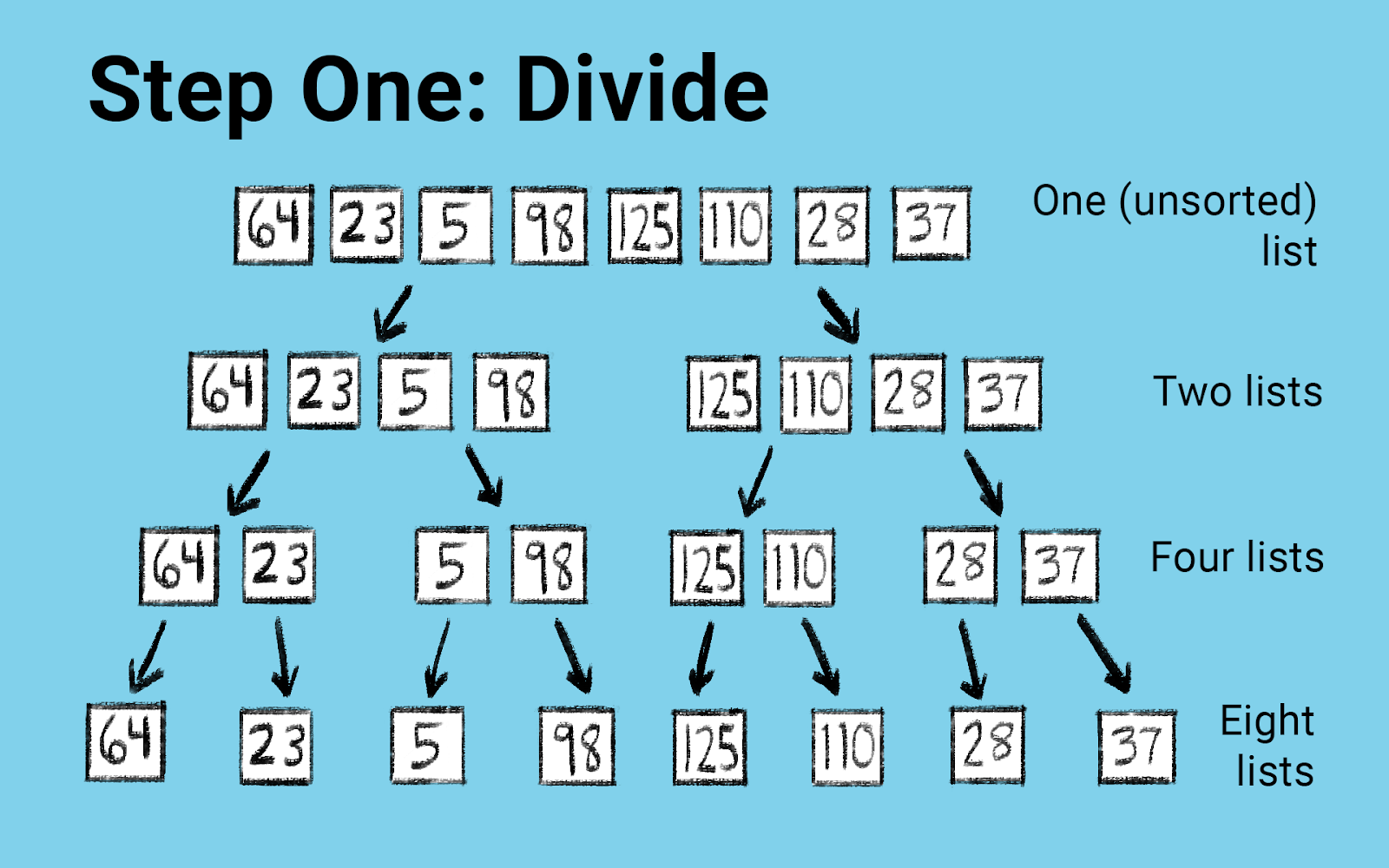Step One: Divide diagram

SAY:

• Looking at this diagram, we’re going to take the cups in front of us, and if we do the subdivisions illustrated in the diagram, we will end up with the eight cups separated out [shown in the row “Eight lists”].

[Separate the eight cups out.]

SAY:

• Now, instead of a list of eight items (i.e., numbers), we end up with eight lists of one item each.

• Each of these lists of one item is sorted because they only have one number in them.

• We have subdivided the problem into the smallest parts possible.

SAY:

• The second part of the merge sort is “conquer.”

[Project the Merge Sort — Step Two: Conquer diagram on a projection screen.]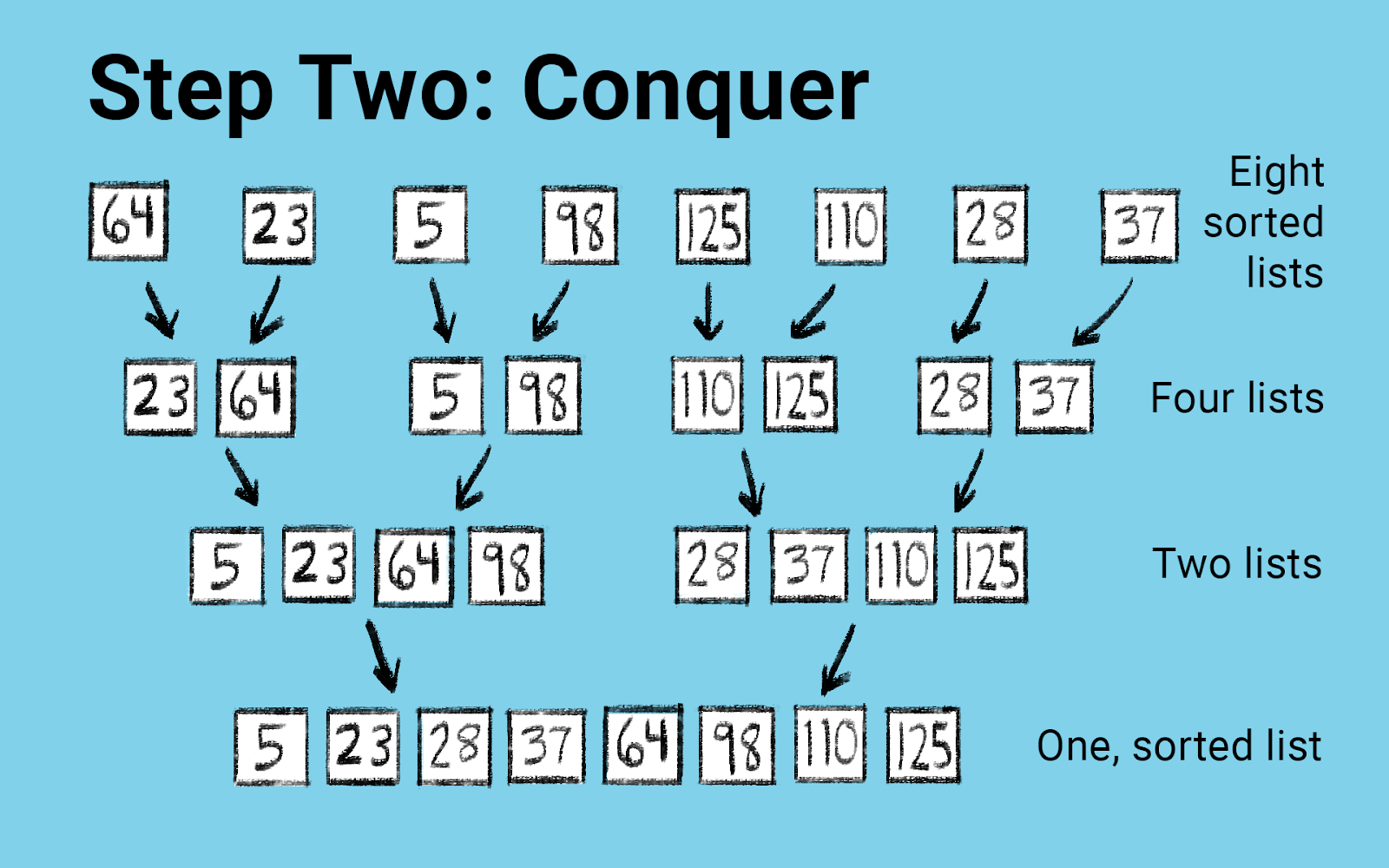Step Two: Conquer diagram

SAY:

• We see from the diagram that we first take the eight sorted lists and merge them into four ordered pairs.

• Let’s demonstrate this with the first two cups.

• We merge the single numbered list 64 with the single numbered list 23 by first taking the smaller numbered cup, 23, out. [Move the cup numbered 23 in front of the cup numbered 64.]

• Then, what remains is the single numbered list of 64. We take this cup out and place it next to 23. [Then, place the cup numbered 64 next to the cup numbered 23.]

SAY:

• We’ll repeat this process for the remaining cups.

• If we continue in this way, we’ll end up with four lists where each of the lists are ordered.

[Place the cups in the arrangement provided in the “Four lists” row of the Merge Sort — Step Two: Conquer diagram]

SAY:

• Now, we’ll merge the first two ordered pairs to create a single list of four ordered numbered cups.

• Let me show you how we do this.

• First, we look at the first cups in each pair (23, and 5). We take out the smallest numbered cup. In this case, it will be the cup numbered 5. [Place this numbered cup out in front of the other cups.]

• Then, in the remaining two lists [the list with 23, and the list with 5 and 98 [note the 5 was removed in the previous step], we again look at the first cup in each of these two lists.

• We’ll pull out the cup with the smaller number. In this case, it’s 23. [Take the 23 out, and place it next to the cup numbered 5.]

• Now, we’re left with two lists with one number in each.

• We’ll take the smaller number from these two lists, which is 64, and place it next to 23. [Take the 64 out, and place it next to the cup numbered 23.]

• Finally, we’ll place the last numbered cup, 98, next to 64. [Take the 98 out, and place it next to the cup numbered 64.]

SAY:

• Now, we have a list of four numbered cups that are ordered.

• Then, we would repeat the same process with these two pairs of cups. [Point to the pairs of cups numbered 110 and 125, and 28 and 37.]

[Do not actually go through the process. Instead, indicate what the end result would look like by pointing to the way the cups numbered 110 and 125, and 28 and 37 would be merged, provided in the “Two lists” row of the Merge Sort — Step Two: Conquer diagram]

SAY:

• Finally, as shown in the diagram, we have two sorted lists. Now, we’ll merge these two lists. The approach will be the same one we used earlier (where we merged four lists into two lists). [Point to the “Four lists” row and the “Two lists” row of the Merge Sort — Step Two: Conquer diagram.]

• Our list of numbered cups are now ordered! [Point to the “One, sorted list” row of the Merge Sort — Step Two: Conquer diagram.]

• The problem is solved!

SAY:

• This merge sort involved 15 comparisons, versus 22 comparisons in our bubble sort example.

• The merge sort wins!

• When executing the bubble sort and merge sort on a computer for a list of thousands of numbers, the merge sort takes fractions of a second, while the bubble sort’s running time is in minutes. The merge sort is significantly more efficient than the bubble sort.

SAY:

• In this activity, we saw two ways, out of many, that computers sort data.

• It is not uncommon for multiple algorithms to accomplish the same task. However, some algorithms are faster and more efficient than others, as we saw in the case of the divide and conquer merge sort, versus the simple but time-consuming bubble sort.

• Sorting algorithms are an important family of computer algorithms with many useful applications.

• Other families of algorithms include search algorithms, route-finding algorithms used by GPS systems, and cryptography algorithms that secure messages over the Internet.

• In recent years, you may have heard of, and very likely encountered, AI machine learning algorithms.

• These algorithms depart from traditional programmable computer algorithms.

• What is significant about AI machine learning algorithms is that they learn (train) on their own from data, and a lot of it, with the goal of optimizing their responses.

• AI machine learning happens when Facebook tags your photos, Siri or Alexa answers your questions, and when self-driving cars appear on the roadways, to name just a few instances.

Activity #3: Algorithms in Prime Time

SAY:

• Let’s look at a problem that has pushed the boundaries of algorithmic thinking for over 2,000 years — finding prime numbers.

• As a reminder, a prime number is just an integer greater than 1 that is divisible only by 1 and itself. For example, 2, 3, 5, 7, and 11 are prime numbers.

• The number 6, for instance, is not a prime, since 6 is divisible by 1, 2, 3, and 6. The number 15 is also not a prime — it’s divisible by 1, 3, 5, and 15.

• Prime numbers are, in fact, the building blocks of our number system, and today, prime numbers play an important role in cryptography and Internet security.

• Securing transactions across the Internet, like purchasing items with a credit card, rely on encryption (i.e., the science of encoding messages) with very large primes — prime numbers with hundreds or even thousands of digits.

SAY:

• Although there are an infinite number of primes, finding very large primes is quite difficult.

• Let’s look at a simpler problem — finding all primes up to 100, with a fun exercise you’ll do in pairs.

[Pass out the Algorithms in Prime Time: Participant Handout.]

SAY:

• Let’s represent integers up to 100 in increasing order using the 10 by 10 array shown on your handout.

• Cross out 1 and then cross out all even numbers — multiples of 2 (i.e., numbers divisible by 2) — except for 2. Then, circle 2, which is a prime.

• What do you think the next step should be?

[Cross out all multiples of 3 (i.e., numbers divisible by 3), except 3. Circle 3, another prime number. Note that some numbers might already have been crossed out, such as 6 (which is a multiple of both 2 and 3).]

SAY:

• Now, working in pairs, I want you to take the next 15 minutes to identify all remaining prime numbers up to 100. As you do so, on your handout, write out the steps you’re using to identify these prime numbers. Make sure to begin your algorithm by writing down the steps we just discussed together (e.g., first, representing the integers from 1 to 100 in a 10 by 10 array in increasing order).

• You want to write an algorithm so that if you were to give it to any of your friends, they would be able to (without your help) follow the steps to find all primes up to 100.

• To begin, first, write your goal on the handout.

• After you write the steps to your algorithm, also indicate the input and outputs of your algorithm below.

• There are a few things I’d like you to keep in mind as you do this exercise.

• Is your algorithm allowing you to identify all primes up to 100? In other words, is it achieving its goal?

• Are there ways you could make your algorithm more efficient?

[Organize participants into pairs. Allow 15 minutes for this exercise.]

SAY:

• Let’s come back together. Here is one way to express the steps of this algorithm.

[Project the algorithm on the Algorithms in Prime Time: Educator Handout (starting from the “Goal” and ending with “This algorithm is known as the Sieve of Eratosthenes.”) on a projection screen. Keep this projection up until you reach the next projection, of a Python code of the Sieve of Eratosthenes.]

SAY:

• The algorithm that you have just written is a very famous one.

• It is known as the Sieve of Eratosthenes, developed by the Greek mathematician Eratosthenes, and dates back to 240 B.C.

• How do you know that the algorithm you developed in groups identifies all primes up to 100?

[A possible answer might be that participants have identified all numbers that are multiples of only 1 and themselves (i.e., these numbers are divisible by only 1 and themselves), and circled these numbers.]

• In your pairs, did you find ways to make this algorithm more efficient?

[A possible answer might be that once participants reached the prime number 11, they noticed that all of its multiples have been crossed out. For example, 44 was crossed out as a multiple 2. And 33 was crossed out as a multiple of 3. Furthermore, once we reach 11 x 11, we notice that the product (121) is greater than 100. We can then circle all the remaining numbers (i.e., the numbers that haven’t already been crossed out), all of which are primes.]

SAY:

• We can modify this fourth step [point to the fourth step on the Algorithms in Prime Time: Educator Handout] to indicate that we can stop at the number 11, the first prime whose square (i.e., the product of 11 x 11) is greater than 100.

[Then, on the Algorithms in Prime Time: Educator Handout, point to the input of 100.]

• Is the input of 100 correct?

[Yes. As the goal of the algorithm indicates, we are aiming to find all primes up to 100.]

SAY:

• We can actually input any positive integer and modify the algorithm accordingly to find all prime numbers up to that integer.

SAY:

• Let’s suppose instead of inputting 100, our input is 1,000.

• We could use the Sieve of Eratosthenes to find all prime numbers up to 1,000 by paper and pencil. This would take us quite a long time.

• But, a computer could execute the steps of this algorithm almost instantaneously!

SAY:

• The Sieve of Eratosthenes written in the programming language Python (or in any programming language) is able to print out all primes up to 1,000 in a fraction of a second.

[Project the following Python code on a projection screen.]

======

def apply_sieve(n):

# n is the size of the sieve

# Key Idea:

#  If a[i] == 0, then number i has been "crossed out",

#  if a[i] == 1, then the number i is not (yet) crossed out.

a = *(n+1)  # Start with a list of 1s, of length (n+1).

a = 0  # set to zero, as

a = 0  # neither 0 nor 1 are primes

p = 2  # 2 is the first prime

pmax = int(round(n**0.5)) + 1  # we only need to sieve up to square         root of n.

while p < pmax:

while a[p] == 0:

p += 1

j = 2*p

while j < n:

a[j] = 0

j += p

p += 1

# return the list of primes, which are the numbers we have     NOT crossed out.

return [p for p in range(n) if a[p] == 1]

N = 1000  # Look for primes in the first one hundred numbers.

primes = apply_sieve(N)

print(primes)

>> 2,3,5,7,11,13,17,19,23,29,31,37,41,43,47,53,59,61,67,71,73,79,83,89,97

=========

SAY:

• But, if you try to find very large prime numbers — primes with hundreds or thousands of digits, which play an important role in cryptography and Internet security — the computer algorithm of the Sieve of Eratosthenes becomes very inefficient.

• Over many decades, mathematicians, computer scientists, and engineers have developed a wide collection of methods / algorithms to identify these very large primes — some of which are variations of the Sieve of Eratosthenes, and others find inspiration from probability theory. Even molecular chemistry has come into play!

Assignment

SAY:

• Now that we’ve talked about what an algorithm is (and isn’t!), and illustrated several different algorithms, I want you to apply what you’ve learned to create your own algorithm.

• This algorithm can be related to any topic you’d like — a set of steps that solve a math problem, or maybe even the steps that accomplish an action in your favorite mobile application or video game.

• Before you write down the steps, make sure, at the top, you specify your goal. Then, write the steps for the given algorithm. Your algorithm should be between five and 10 steps. Make sure to also write the input(s) and output(s) of your algorithm!

• As you develop your algorithm, keep in mind the key characteristics of an algorithm that we discussed earlier. That an algorithm’s instructions are well-defined, the algorithm accomplishes your goal, and (though not required), ideally, that it’s efficient.

[Give participants 25 minutes to complete the activity. Depending on the time allotted, in the current or the second group convening, engage in the discussion below.]

Discussion

[Organize participants into pairs.]

SAY:

• In pairs, I want you to share your algorithm, and discuss:

• Do you think that you could replicate the steps of your partner’s algorithm? If not, what modifications might you suggest?

• Do you think there are ways to make your partner’s algorithm more efficient? If so, how?

• Was there anything new or surprising that you each learned while creating your own algorithm, or reading your partner’s algorithm?

• You will have 15 minutes to complete this exercise.

[Give participants 15 minutes to engage in this exercise with their partner. Afterward, allow 10 minutes for pairs to share out a) any ways they can make their algorithm more efficient, based on their discussion with their partner, and / or b) a new or surprising thing they have learned while developing their own algorithm, or reviewing their partner’s algorithm.]

Individualmente o en grupo
En grupo
Fecha de publicación
July, 2022
Idiomas
Inglés